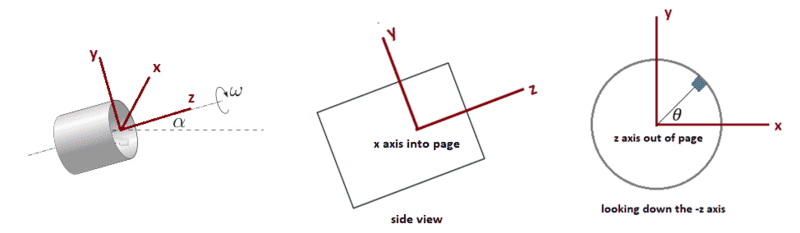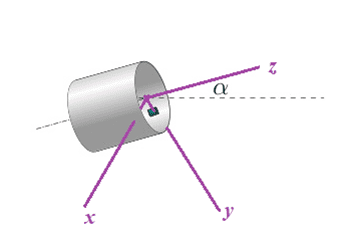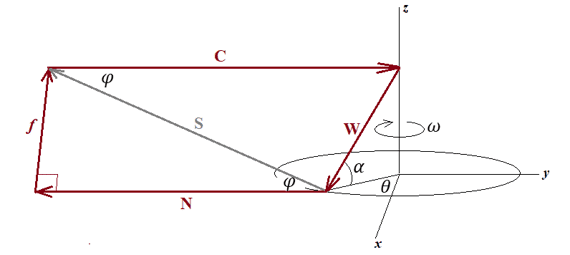# Block in a rotating cylinder

A vector ##\vec{u}## is defined by ##(\vec{u_{x}}, \vec{u_{y}}, \vec{u_{z}})##

TSny
Homework Helper
Gold Member
A vector ##\vec{u}## is defined by ##(\vec{u_{x}}, \vec{u_{y}}, \vec{u_{z}})##
Yes. But the values of the components depend on the orientation of the axes. So, you need to describe the orientation of your choice of the x, y, and z axes.

One thing that must be true is that ##g^2 = g_x^2 + g_y^2 + g_z^2##. Is this true for your expression ##\vec{g} = (g cos \theta, g sin \theta, g cos \alpha)##?

Last edited:
A vector ##\vec{u}## is defined by ##(\vec{u_{x}}, \vec{u_{y}}, \vec{u_{z}})##
I'd suggest working in cylindrical coordinates.

Yes. But the values of the components depend on the orientation of the axes. So, you need to describe the orientation of your choice of the x, y, and z.

z is oriented as the axe of rotation of the cylinder if ##\alpha=0##
y is oriented towards the axe of rotation (still if ##\alpha=0##)
x by consequence

I'd suggest working in cylindrical coordinates.

In cylindrical coordinates the axe ##z=\hat{z}##, but I must direct the ##\hat{z}## in the direction of the axe of rotation (inclined by an angle ##\alpha##)?

TSny
Homework Helper
Gold Member
z is oriented as the axe of rotation of the cylinder if ##\alpha=0##
y is oriented towards the axe of rotation (still if ##\alpha=0##)
x by consequence
OK, I'm not sure I follow your description of the y axis. Suppose you pick the axes as shown below for ##\alpha \neq 0##. The z axis is the axis of the cylinder and the yz plane is vertical. The x axis is then horizontal.How would the components of ##\vec{g}## be expressed in this coordinate system?

When the block is at the angle ##\theta## shown, what would be a unit vector in the centripetal direction?

Use these vectors to find the component of ##\vec{g}## that is normal to the surface when the block is in this position.

Last edited:
hi all I happen to be doing this same problem I am having some problem for part b can I ask it here thaks

Last edited:
TSny
Homework Helper
Gold Member
hi all I happen to be doing this same problem I am having some problem for part b can I ask it here thaks
I think that would be OK. The OP, @fedecolo , does not appear to be seeking any more help regarding this problem.

@TSny
thanks and sorry for the late reply
here is my solution to part a help me check if it is correct it is similar to zweirz's solution

a)
##
f_r = 0 = N - mgsin\theta - mw^2 r\\
N = mgsin\theta +mw^2 r\\
f_\theta = 0 = F - mg cos \theta \\
F = mg cos \theta <= \mu N\\
cos \theta + sin \theta \mu <= \mu \frac{m w^2 r}{ g}\\
##
eyeballing the problem the normal force is clearly least in the first quadrant so the signs are correct
taking derivatives setting zero solving for sin and cos got me
##
\sqrt {\mu ^-2 + 1 } <= \frac{m w^2 r}{g}
##

I tried applying the same procedure for part b I decided to work with cylindrical coordinates with z axis being similar to your and r and theta axis being the usual one along the plane of cylinder

##
g_z = g sin \alpha\\
g_{r,\theta} = g cos \alpha \\
##
so the radial equation is the same as before except g changes
##
f_r = 0 = N + mg cos\alpha sin \theta = m w^2 r\\
N =m w^2 r - mg cos\alpha sin \theta \\
##
now there is two components of friction F_theta and F_z whose comined modulus must be less than \mu N
##
F_\theta = mg cos \alpha cos \theta\\
F_z = mg sin \alpha \\
\sqrt { F_\theta ^2 + F_z ^2}\\
\sqrt {(cos \alpha cos \theta)^2 + sin \alpha ^2} <= \mu(\frac{w^2 r}{g} - cos \alpha sin \theta)\\
cos \alpha(\sqrt {(cos \theta)^2 + tan ^2\alpha } + \mu sin \theta)<= \mu(\frac{w^2 r}{g} )\\
##
after long differentiating I am getting
##
sin \theta = \frac{\mu sec \theta}{\sqrt{\mu^2 + 1}}\\
cos \theta = \sqrt{\frac{1 - \mu^2 tan^2 \alpha }{\mu ^2 +1}}\\
##
plugging back in I get exactly same answer as first part of the problem what am I doing wrong

TSny
Homework Helper
Gold Member
I noticed a couple of typographical errors for part (a) which did not affect your subsequent work:
## cos \theta + sin \theta \mu <= \mu \frac{m w^2 r}{ g}##
##\sqrt {\mu ^-2 + 1 } <= \frac{m w^2 r}{g}##
There should not be a factor of ##m## on the right side of the above equations.

Otherwise, the rest of your work looks good except that you need to deal with the following important situation in part (b):

after long differentiating I am getting
##
sin \theta = \frac{\mu sec \theta}{\sqrt{\mu^2 + 1}}\\
cos \theta = \sqrt{\frac{1 - \mu^2 tan^2 \alpha }{\mu ^2 +1}}\\
##
What happens if the quantity inside the square root is negative?

•timetraveller123
ok then for the case when
##
\mu <= cot \alpha
##
that's the answer but I don't really know what to do if the term becomes negative what could it possibly represent and mean

TSny
Homework Helper
Gold Member
if the term becomes negative what could it possibly represent and mean
The expression ##\cos \theta = \sqrt{\frac{1 - \mu^2 tan^2 \alpha }{\mu ^2 +1}}## arose from setting the derivative of some function ##f(\theta)## equal to zero . What if the function doesn't have any point where its derivative is zero?

•timetraveller123
oh so is the function i was trying to maximize monotonically increasing in the interval 0 < theta<pi/2 and only reaches maximum where cos x is not defined hence the maximum in the interval is at pi/2 is it that
so for that case the maximum is reached with cos being real is angle pi/2 is it? assuming so

so is it ##
\omega^2 >=\frac{g}{r}(\frac{sin \alpha}{\mu} + cos \alpha)
##

TSny
Homework Helper
Gold Member
Yes, I believe that's right. You might check the special case where ##\alpha = \pi/2##.

•timetraveller123
Yes, I believe that's right. You might check the special case where ##\alpha = \pi/2##.
oh ya it reduces to
##
mg = N \mu
##
just out of curiosity why is the answer same as the first part if ##
\mu <= cot \alpha
##
what does it physically represent

TSny
Homework Helper
Gold Member
oh ya it reduces to
##
mg = N \mu
##
just out of curiosity why is the answer same as the first part if ##
\mu <= cot \alpha
##
what does it physically represent
I was surprised, too, when I first saw that the answer for ##\omega_{\rm min}## is independent of ##\alpha## as long as ##\mu \le \cot \alpha##. I don't see a nice physical argument for this.

The mathematical reason becomes clearer if you first show that for any ##\alpha##, the friction force ##f## and the normal force ##N## may be written as

##f = mg\sqrt{1-\beta^2} \,\,\,\,## and ##\,\,\,\, N = m\omega^2 R - mg \beta \,\,\,## where ##\beta## is defined by ##\beta = \cos \alpha \sin \theta##.

Then, ##\mu N \ge f## leads to

##\large \frac{\mu R \omega^2}{g}## ##\ge \mu \beta + \sqrt{1-\beta^2}##.

Thus, the minimum value of ##\omega## is determined by the maximum value of the right hand side of this relation over the domain of allowed values of ##\beta##. The only parameter on the right hand side is ##\mu##. So, you would expect that the maximum value of the right hand side depends only on ##\mu## and not on ##\alpha##.

However, there is a complication due to the fact that ##\beta## is limited to a maximum value of ##\cos \alpha## (since ##\beta = \cos \alpha \sin \theta##). Thus, you have the possibility that the maximum value of the right hand side of the above relation occurs at this upper limit of ##\beta## where ##\beta = \cos \alpha##. In this case, ##\omega_{\rm min}## depends on both ##\mu## and ##\alpha##, as you stated in post #38. This occurs whenever ##\mu \ge \cot \alpha##.

Last edited:
•timetraveller123
@TSny
once again as usual sorry for insanely late reply didn't mean to be rude and forgot to thank you
thanks for the help i get it now

Sorry about bumping the problem, but apparently there is a different approach to part a and b using geometry. In part a it is clear that we have A fixed plane containing the vector g and vector w^2.R. In the reference frame rotating with the block we can see that the resulting field of these two vectors points in a circle (since vector g rotates) with that idea and some ideas about the friction and normal forces we can do letter a. Now my question is about letter b. Since the plane is moving with time, I can't see geometrically how things happen between vectors g and w^2.R. Only thing I know is that, because in reference frame rotating block is at rest, the forces mg, mw^2.R and the resultant of normal and friction form a triangle. So indeed they are at the same plane at each instant.

TSny
Homework Helper
Gold Member
,
Sorry about bumping the problem, but apparently there is a different approach to part a and b using geometry. In part a it is clear that we have A fixed plane containing the vector g and vector w^2.R. In the reference frame rotating with the block we can see that the resulting field of these two vectors points in a circle (since vector g rotates) with that idea and some ideas about the friction and normal forces we can do letter a.
It took me a while to grasp what you are saying here. I think I got it. This graphical construction is a nice way to get to the answer.

Now my question is about letter b. Since the plane is moving with time, I can't see geometrically how things happen between vectors g and w^2.R. Only thing I know is that, because in reference frame rotating block is at rest, the forces mg, mw^2.R and the resultant of normal and friction form a triangle. So indeed they are at the same plane at each instant.
Yes, the graphical construction of the force diagram in the frame rotating with the cylinder for part b is more complicated than part a. I spent a lot of time on this. But once constructed, the diagram can be used to solve part b without a lot of calculation.

I'm not sure if my diagram is similar to yours. I chose an x-y-z coordinate system rotating with the cylinder as shown:In the rotating frame of the cylinder, the block and the x-y-z axes remain fixed with the y-axis passing through the block.

The forces acting on the block are shown added together in the force diagram below where I reoriented the diagram so that the x-y plane is oriented horizontally in the figure.The normal force N and the centrifugal force C are always parallel to the y-axis. In this orientation of the figure, the axis of the cylinder (z-axis) is vertical. In this frame of reference, the x, y, and z axes remain fixed while the vector representing the weight W rotates around the z-axis with angular speed ##\omega##. Thus, W sweeps out a cone with the tip of the W vector tracing a circle. W maintains a constant angle ##\alpha## to the x-y plane, where ##\alpha## is the amount of "tipping up" of the cylinder in the first diagram above. The friction force f varies in time so that these four vectors (f, C, W, and N) always add to zero. (The block is in static equilibrium in this frame.)

The time-dependent vector S is defined as the sum of the forces N and f.
##\theta## gives the instantaneous angle that the tip of W has rotated from the x-axis.
##\varphi## is the angle shown in the triangle formed by f, N, and S. ##\varphi## varies with time.

Is this similar to the diagram that you had in mind?

Note that the block is on the verge of slipping if ##\tan \varphi = \mu##. For the block to not slip, we must have ##\varphi \leq \arctan \mu## for all positions of W as W sweeps out its cone. Using this idea along with the geometry of the figure, you can deduce the minimum value of ##\omega## for which the block will not slip.

•JD_PM and Moara
Actually no, since at all times the three vectors W,C and R (where R is resultant of N and friction) are in a plane I am trying to fix this plane (although it changes in time ) and figure out what type o figure W+C makes. It appears to me is going to be similar to letter a, but instead of a circle, it might be an arc of a circle, but I couldn't find an easy way to show this.

TSny
Homework Helper
Gold Member
Actually no, since at all times the three vectors W,C and R (where R is resultant of N and friction) are in a plane I am trying to fix this plane (although it changes in time ) and figure out what type o figure W+C makes. It appears to me is going to be similar to letter a, but instead of a circle, it might be an arc of a circle, but I couldn't find an easy way to show this.
Your vector R is the same as my vector S. (I didn't want to use R, since this symbol is also used for the radius of the cylinder.) At any instant of time, all five vectors N, C, W, f , and S lie in one plane. The orientation of this plane changes with time. For example, when ##\theta = \pi/2## or ##3 \pi/2## the plane is vertical in the figure of my previous post.

It all seems complicated, but the only thing that we are really interested in is the maximum value of angle ##\varphi##. If ## \tan \varphi## exceeds ##\mu##, the block will slip.

The magnitudes of C and W remain constant in time. With that in mind, if you wanted to maximize ##\varphi## in the triangle CWS, how would you want W and S to be oriented relative to each other?

As zwierz did, I have ##N=m \omega^2 R-mg sin \theta ## and ## N= \frac{mg cos \theta}{\mu}## and then ##\mu \geq \frac{g cos \theta}{\omega ^2 R-g sin \theta}## and so ## \omega^2 R \geq g sin \theta ## because the denominator must be > 1 (otherwise the fraction would be > 1 and the block would slide)

Why Is the normal force in this case not just ##N=mg sin\theta## ?? This is already the component of mg which is perpendicular to the surface. And since you have written ## N= \frac{mg cos \theta}{\mu}##, then I guess the frictional force is ## mg cos \theta##. Why is the frictional force the component of mg which is parallel to the surface?

TSny
Homework Helper
Gold Member
Why Is the normal force in this case not just ##N=mg sin\theta## ??
The normal force is found by using Newton's 2nd law in the radial direction: ##\sum F_r = ma_r##. What is ##a_r## for circular motion?

Why is the frictional force the component of mg which is parallel to the surface?
Apply Newton's second law in the tangential direction: ##\sum F_t = ma_t##. The block moves in uniform circular motion. So, what can you say about ##a_t##?